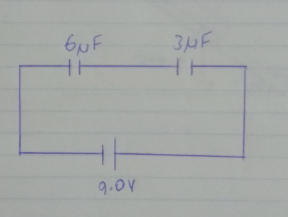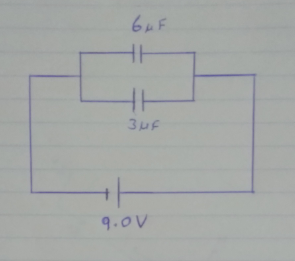# Problem: You have two capacitors, one is 6.0 μF the other is 3.0 μF. You also have some wires and a 9.0 V battery. a) Using the schematic symbols shown later in the lab, draw a diagram of a circuit with the two capacitors connected in series with the battery. Draw a diagram of a circuit using the same battery and capacitors with the capacitors now connected in parallel. Find the equivalent capacitance for each circuit.

###### FREE Expert Solution

a)

In series:In parallel:90% (205 ratings)###### Problem Details

You have two capacitors, one is 6.0 μF the other is 3.0 μF. You also have some wires and a 9.0 V battery.

a) Using the schematic symbols shown later in the lab, draw a diagram of a circuit with the two capacitors connected in series with the battery. Draw a diagram of a circuit using the same battery and capacitors with the capacitors now connected in parallel. Find the equivalent capacitance for each circuit.